# Probability-NCERT Solutions

Class X Math
NCERT Solution for Probability
EXERCISE 15.1
Q.1.   Complete the following statements:
(i) Probability of an event E + Probability of the event ‘not E’ + ............ .
(ii) The probability of an event that cannot happen is ............. . Such an event is called ........... .
(iii) The probability of an event that is certain to happen is ................ . Such an event is called ................
(iv) The sum of the probabilities of all the elementary events of an experiment is................ .
(v) The probability of an event is greater than or equal to................ and less than or equal to ................ .
Sol. (i) Probability of an event E + Probability of the event not E = 1.
(ii) The probability of an event that cannot happen is 0. Such an event is called impossible event.
(iii) The probability of an event that is certain to happen is 1. Such an event is called sure or certain event.
(iv) The sum of the probabilities of all the elementary events of an experiment is 1.
(v) The probability of an event is greater than or equal to 0 and less than or equal to 1.
Q.2.   Which of the following experiments have equally likely outcomes? Explain.
(i) A driver attempts to start a car. The car starts or does not start.
(ii) A player attempts to shoot a basketball. She/he shoots or misses the shot.
(iv) A baby is born. It is a boy or a girl.
Sol. (i) Since, the car may or may not start, thus the outcomes are not equally likely.
(ii) The player may shoot or miss the shot.
∴ The outcomes are not equally likely.
(iii) In advance it is known that the answer is to be either right or wrong.
∴ The outcomes right or wrong are equally likely to occur.
(iv) In advance it is known the newly bom baby has to be either a boy or a girl.
∴ The outcomes either a boy or a girl are equally likely to occur.
Q.3.   My is tossing a coin considered to be a fair way of deciding which team should get the ball at the beginning of a football game?
Sol. Since on tossing a coin, the outcomes 'head' and 'tail' are equally likely, the result of tossing a coin is completely unpredictable and so it is a fair way.
Q.4.   Which of the following cannot be the probability of an event?
(A)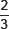(B) –1.5
(C) 15%
(D) 0.7
Sol. Since, the probability of an event cannot be negative,
∴ (B) –1.5 cannot be the probability of an event.
Q.5.   If P(E) = 0.05, what is the probability of 'not E'?
Sol. ∵ P(E) + P(not E) = 1
∴ 0.05 + P(not E) = 1 ⇒ P(not E) = 1 – 0.05
= 0.95
Thus, probability of 'not E' = 0.95.
Q.6.   A bag contains lemon flavoured candies only. Malini takes out one candy without looking into the bag. What is the probability that she takes out
(i) an orange flavoured candy?
(ii) a lemon flavoured candy?
Sol. (i) Since, there are lemon flavoured candies only in the bag,
∴ Taking out any orange flavoured candy is not possible.
⇒ Probability of taking out an orange flavoured candy = 0.
(ii) Also, probability of taking out a lemon flavoured candy = 1.
Q.7.   It is given that in a group of 3 students, the probability of 2 students not having the same birthday is 0.992. What is the probability that the 2 students have the same birthday?
Sol. ∴ Let the probability of 2 students having same birthday = P(SB)
And the probability of 2 students not having the same birthday = P(nSB)
∴ P(nSB) + P(nSB) = 1
⇒ P(SB) + 0.992 = 1
⇒ P(SB) = 1 – 0.992 = 0.008
So, the required probability of 2 boys having the same birthday = 0.008.
Q.8.   A bag contains 3 red balls and 5 black balls. A ball is drawn at random fret the bag. What is the probability that the ball drawn is (i) red? (ii) not red?
Sol. Total number of balls = 3 + 5 = 8
∴ Number of all possible outcomes = 8
(i) For red balls:
∵There are 3 red balls.
∴ Number of favourable outcomes = 3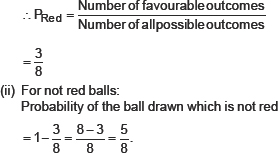Q.9.   A box contains 5 red marbles, 8 white marbles and 4 green marbles. One marble is taken out of the box at random. What is the probability that the marble taken out will be (i) red? and (ii) white?
(iii) not green?
Sol. Total number of marbles = 5 + 8 + 4 = 17
(i) For red marbles:
∵Number of red marbles = 5
∴ Number of favourable outcomes = 5
∴ Probability of red marbles, P(red)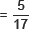(ii) For white balls:
∵Number of white balls = 8
∴ Probability of white balls,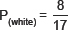(iii) For not green balls:
∵Number of white balls = 4
∴ Number of 'not green' balls = 17 – 4 = 13
i.e., Favourable outcomes = 13
∴ Probability of ball 'not green'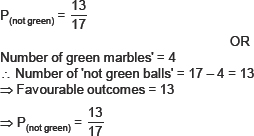Q.10.   A piggy bank contains hundred 50p coins, fifty Re 1 coins, twenty Rs. 2 coins and ten Rs. 5 coins. If it is equally likely that one of the coins walla out when the bank is turned upside down, what is the probability that the coin (i) will be a 50p coin? and (ii) will not be Rs. 5 coin?
Sol. Number of:
50 p coins = 100
Re 1 coins = 50
Rs 2 coins = 20
Rs 5 coins = 10
Total number of coins = 100 + 50 + 20 + 5 = 180
(i) For a 50 p coin:
Favourable events = 100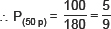(ii) For not a Rs. 5 coin:
∵ Number of Rs. 5 coins = 10
∴ Number of 'not Rs. 5' coins = 180 – 10 = 170
⇒ Favourable outcomes = 170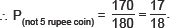Q.11.   Gopi buys a fish from a shop for his aquarium. The shopkeeper take out one fish at random from a tank containing 5 male fish and 8 fernale fish (see Fig.). What is the probability that the fish taken out is a male fish?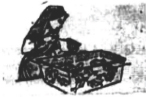Sol. Number of:
Male fishes = 5
Female fishes = 8
∴ Total number of fishes = 5 + 8 = 13
⇒ Total number of outcomes = 13
For a male fish:
Number of favourable outcomes = 5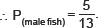Q.12.   A game of chance consists of spinning an arrow which comes to rest pointing at one of the numbers 1, 2, 3, 4, 5, 6, 7, 8 (see figure), and these are equally likely outcomes. What is the probability that it will point at
(i) 8?                (ii) an odd number?
(iii) a number greater than 2?
(iv) a number less than 9?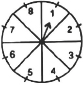Sol. Total numbers marked = 8
(i) When pointer points at 8:
Total number of outcomes = 8
Number of favourable outcomes = 1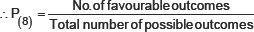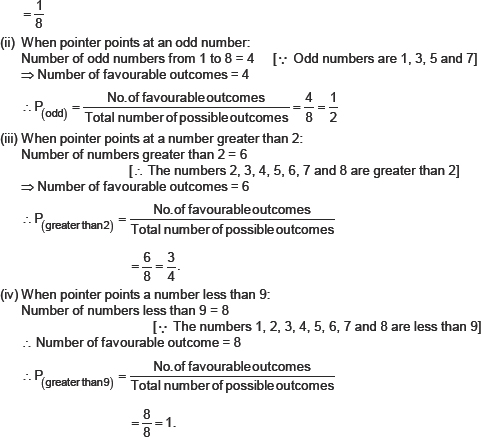Q.13.   A die is thrown once. Find the probability of getting:
(i) a prime number; (ii) a number lying between 2 and 6; (iii) an odd number.
Sol. Since, numbers on a die are 1, 2, 3, 4, 5, and 6.
∴ Number of total outcomes = 6
(i) For prime numbers:
Since 2, 3, and 5 are prime number,
∴ Favourable outcomes = 3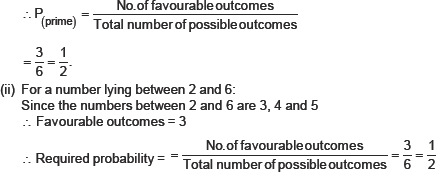(iii) For an odd number:
Since 1, 3 and 5 are odd numbers.
⇒ Favourable outcomes = 3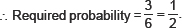Q.14.   One card is drawn from a well-shuffled deck of 52 cards. Find the probability of getting:
(i) a king of red colour
(ii) a face card
(iii) a red face card
(iv) the jack of hearts
(vi) the queen of diamonds
Sol. Number of cards in deck = 52
∴ Total number of possible outcomes = 52
(i) For a king of red colour:
∵Number of red colour kings = 2                                        [∵ Kings of diamond and heart are red]
∴ Number of favourable outcomes = 2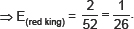(ii) For a face card:
∵4 kings, 4 queens and 4 jacks are face cards
∴ Number of face cards = 12
⇒ Number of favourable outcomes = 12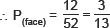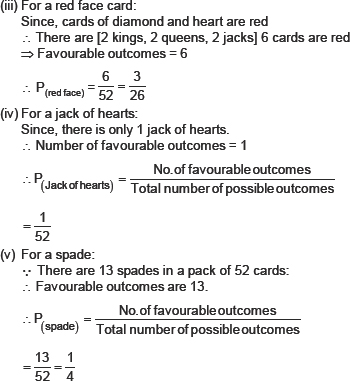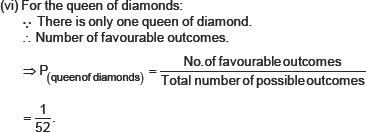Q.15.   Five cards––the ten, jack, queen, king and ace of diamonds, are well-shuffled with their face downwards. One card is then picked up at random.
(i) What is the probability that the card is the queen?
(ii) If the queen is drawn and put aside, what is the probability that the second card picked up is (a) an ace? and (b) a queen?
Sol. We have five cards.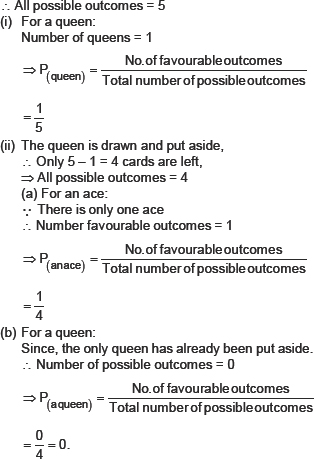Q.16.   12 defectivepens are accidentally mixed with 132 good ones. It is not possible to just look at a pen and tell whether or not it is defective. One pets is taken out at random from this lot. Determine the probability tint the pen taken out is a good one.
Sol. We have
Number or good pens = 132
Number of detective pens = 12
∴ Total number of pens = 132 + 12 = 144
For good pens:
∵ There are 132 good pens
∴ Number of favourable outcomes = 132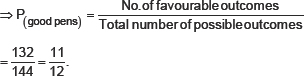Q.17.   (i) A lot of 20 bulbs contain 4 defective ones. One bulb is drawn at random from the lot. What is the probability that this bulb is defective?
(ii) Suppose the bulb drawn in (i) is not defective and is not replaced. Now one bulb is drawn at random fi'otn the rest. What is the probability that this bulb is not defective?
Sol. Since, there are 20 bulbs in the lot.
∴ Total number of outcomes = 20
(i) ∵ Number defective bulbs = 4
i.e., Favourable outcomes = 4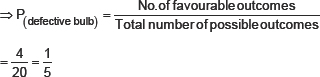(ii) ∵ The bulb drawn above is not included in the lot.
∴ Remaining number of bulbs = 20 – 1 = 19.
⇒ Total number of possible outcomes = 19.
∵ Number of bulbs which are not defective = 19 – 4 = 15
⇒ Favourable number of outcomes = 15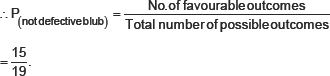Q.18.   A box contains 90 discs which are numbered from 1 to 90. If one disc is drawn at random from the box, find the probability that it bears (i) a two-digit nutner, (ii) a perfect square number and (iii) a number divisible by 5.
Sol. We have:
Total number of discs = 90
∴ Total number of possible outcomes = 90
(i) For a two-digit number:
Since the two-digit numbers are 10, 11, 12,...., 90.
∴ Number of two-digit numbers = 90 -. 9 = 81
[ ∵ 1, 2, 3, 4, 5, 6, 7, 8, and 9 are 1-digit numbers]
⇒ Number of favourable outcomes = 81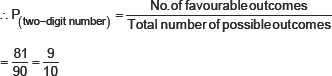(ii) For a perfect square:
Perfect squares from 1 to 90 are 1, 4, 9, 16, 25, 36, 49, 64, and 81
∴ Number of perfect numbers = 9 Number of favourable outcomes = 9
⇒�nNumber of favourable outcomes = 9
(iii) For a number divisible by 5:
Numbers divisible by 5 [from 1 to 90] are: 5, 10, 15, 20, 25, 30, 35, 40, 45, 50, 55, 60, 65, 70, 75, 80, 85, 90
i.e. There are 18 number (1 to 90) which are divisible by 5.
∴ Number of favourable outcomes = 18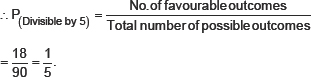Q.19.   A child has a die whose six faces show the letters as given below: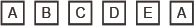The die is thrown once. What is the probability of getting (i) A? and (ii) D?
Sol. Since there are six faces of the given die and these faces are marked with letters∴ Total number of letters = 6
⇒ Number of possible outcomes = 6
(1) For the letter A
∵ Two faces are having the letter A.
∴ Number of favourable outcomes = 2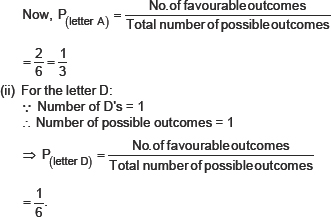Q.20.   Suppose you drop a die at random on the rectangular region shown in figure. What is the probability that it will land inside the circle with diameter 1 m?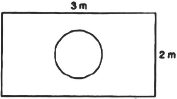Sol. Here, Area of the rectangle = 3 m × 2 m = 6 m2
And, the area of the circle = πr2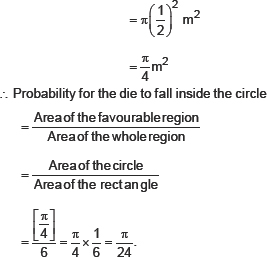Q.21.   A lot consists of 144 ball pens of which 20 are deflective and the others are goad. Nun will buy a pen if it is good, but will not buy if it is deflective. The shopkeeper draws one pen at random and gives it to her. What is the probability that
(ii) She will not buy it?
Sol. Total number of ball pens = 144
⇒ All possible outcomes = 144
(i) Since there are 20 defective pens
∴ Number of good pens 144 – 20 = 124
⇒ Number of favourable outcomes = 124
∴ Probability that she will buy it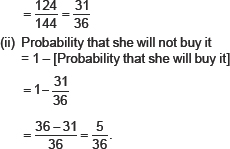Q.22.   Refer to Example 13. (i) Complete the following table: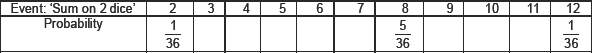(ii) A student argues that th ere are 11 possible sible outcomes 2 , 3, 4, 5, 6, 7, 8 , 9, 10, 11 and 12.
Therefore, each of them has a probability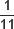. Do you agree with this argument? justify your answer.
Sol. ∵The two dice are thrown together.
∴ Following are the possible outcomes: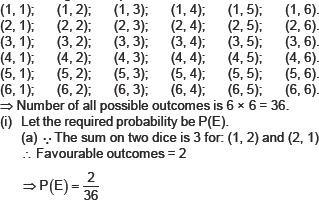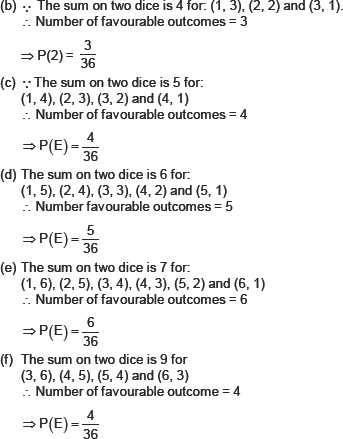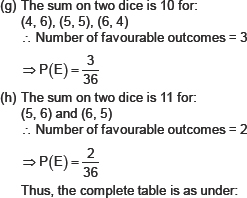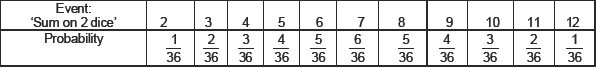(ii) No. The number of all possible outcomes is 36 and not 11.
∴ The argument is not correct.
Q.23.   A game consists of tossing a one rupee coin 3 times and noting its outcome each time. Hanif wins if all the tosses give the same result i.e., three heads or three tails, and loses otherwise. Calculate the probability that Hanif will lose the game.
Sol. Let T denotes the tail and H denotes the head.
∴ All the possible outcomes are:
H H H, H H T, H T T, T T T, T T H, T H T, T T H, H T H
∴ Number of all possible outcomes = 8
Let the event that Hanif will lose the game be denoted by E.
∴ Favourable events are:
H H T, H T H, T H H, T H T, T T H, H T T
⇒ Number of favourable outcomes = 3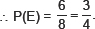Q.24.   A die is thrown twice. What is the probability that
(i) 5 will not come up either time? (ii) 5 will come up at least once?
[Hint: Throwing a die twice and throwing two dice simultaneously are treated as the same experiment.]
Sol. Since, throwing a die twice or throwing two dice simultaneously is the same.
∴ All possible outcomes are: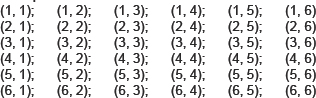∴ All possible outcomes = 36
(i) Let E be the event that 5 does not come up either time, then
The favourable outcomes are [36 – (5 + 6)] = 25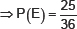(ii) Let N be the event that 5 will come up at least once, then Number of favourable outcomes = 5 + 6 = 11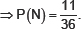Q.25.   Which of the following arguments are correct and which are not correct? Give reasons for your answer.
(i) If two coins are tossed simultaneously there are three possible outcomes-two heads, two tails or one of each. Therefore, fir each of these outcomes, the probability is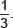(ii) If a die is throum, there are two possible outcomes--an odd number or an even number. Therefore, the probability of getting an odd number is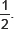Sol. (i) Not correct.
Because, the situation 'one of each' can result in two ways HT and M.
∴ The probability = 4
(ii) Correct.
Because the two outcomes are possible.
EXERCISE 15.2
Q.1.   Two customers Shyam and Ekta are visiting a particular shop in the same week (Tuesday to Saturday). Each is equally likely to visit the shop on any day as on another day. What is the probabilihy that t tlt will visit the shop on (i) the same day? (ii) consecutive days? (iii) different days?
Sol. Here, the number of all the possible outcomes
= 5 × 5 = 25
(i) For both customers visiting same day:
Number of favourable outcomes = 5
[∵ (Tue., Tue.), (Wed., Wed.), (Thu., Thu.), (Fri., Fri.), (Sat., Sat.)]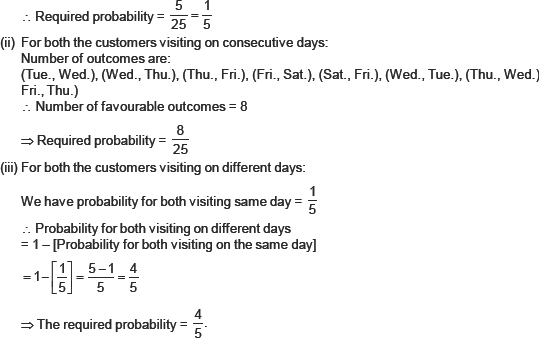Q.2.   A die is numbered in such a way that its faces show the numbers 1, 2, 2, 3, 3, 6. It is thrown two times and the total score in two throws is noted. Complete the following table which gives a fiew values of the total score on the two throws: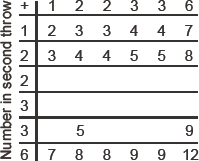What is the probability that the total score is
(i) even?                (ii) 6?                (iii) at least 6?
Sol. The completed table is as under: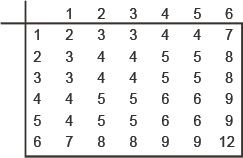∴ Number of all possible outcomes = 36
(i) For total score being even:
Favourable outcomes = 18
[∵ The even outcomes are: 2, 4, 4, 4, 4, 8, 4, 4, 8, 4, 6, 6, 4, 6, 6, 8, 8]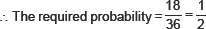(ii) For the score being 6:
In list of score, we have four 6' s.
∴ Favourable outcomes = 4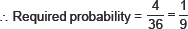(iii) For the score being at least 6:
The favourable scores are:
7, 8, 8, 6, 6, 9, 6, 6, 9, 7, 8, 8, 9, 9 and 12
∴ Number of favourable outcomes = 15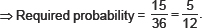Q.3.   A bag contains 5 red balls and some blue balls. If the probability of drawing a blue ball is double that of a red ball, determine the number of blue balls in the bag.
Sol. Let the number of blue balls in the bag be x.
∴ Total number of balls = x + 5
Number of possible outcomes = (x + 5).
For a blue ball favourable outcomes = x
∴ Probability of drawing a blue ball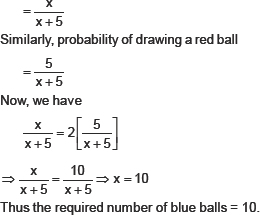Q.4.   A box contains 12 balls out of which x are black. If one ball is drawn at random from the box, what is the probability that it will be a black ball? If 6 more black balls are put in the box, the probability of drawing a black ball is now double of what it was lx f ire. Find x.
Sol. ∵The total number of balls in the box = 12
∴ Number of possible outcomes = 12
Case-I: For drawing a black ball
Number of favourable outcomes = x
∴ Probability of getting a black ball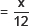Case-II: When 6 more black balls are added
Now, the total number of balls
= 12 + 6
= 18
⇒ Number of possible outcomes = 18
Since, the number of black balls now
= (x + 6).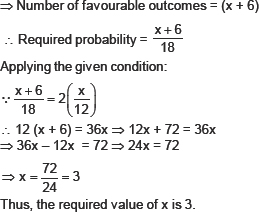Q.5.   A jar contains 24 marbles, some are green and others are blue. If a marble is drawn at random from the jar, the probability that it is green is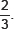Find the number of blue balls in the jar.
Sol. ∵There are 24 marbles in the jar.
∴ Number of possible outcomes = 24.
Let there are x blue marbles in the jar.
∴ Number of green marbles = 24 – x
⇒ Favourable outcomes = (24 – x)
∴ Required probability for drawing a green marble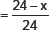Now, according to the condition, we have:Thus, the required number of blue balls is 8.